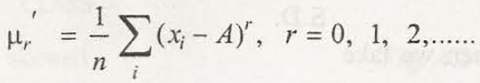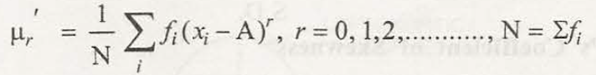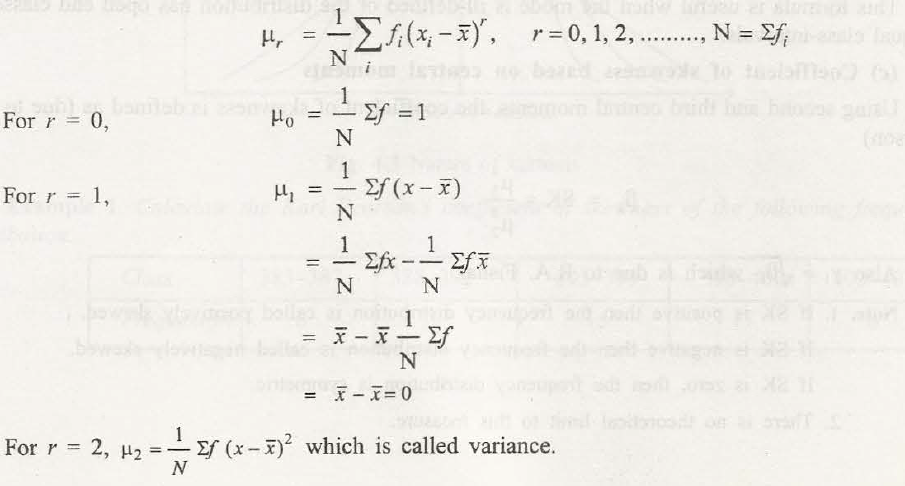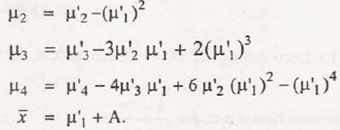The term moment when it comes to statistics can be used in relation to analogous to moments of force. In case of statistics, moments come with constant values that are available in given distribution which can enable to understand nature and form of distribution.

The moments taken from mean are referred by µ. You will get to know that the first four moments are very important which can describe different types of statistical distribution. The formula for first four moments is:

The first moment about the mean, m=

The 2nd moment about the mean, m=

The 3rd moment about the mean, m3 =

The 4th moment about the mean, m4 =

Where,

X = the mid-value of the class

=the mean

f = the class frequency and

N = the total frequency.

Moments that come in mathematical statistics may include basic calculation. Such calculations can also be used to find out probability distribution’s mean, variance and skewness.

First moment

For first moment we set s=1. The formula is said to be:

(x1x2 + x3 + . . . + xn)/nThis can be an identical formula for sample mean.

Second moment

The formula for 2nd moment is:(x12 + x22 + x32 + . . . + xn2)/nThird moment

The formula is:

(x13 + x23 + x33 + . . . + xn3)/n### Customer Reviews

My Homework Help
Rated 5.0 out of 5 based on 510 customer reviews at